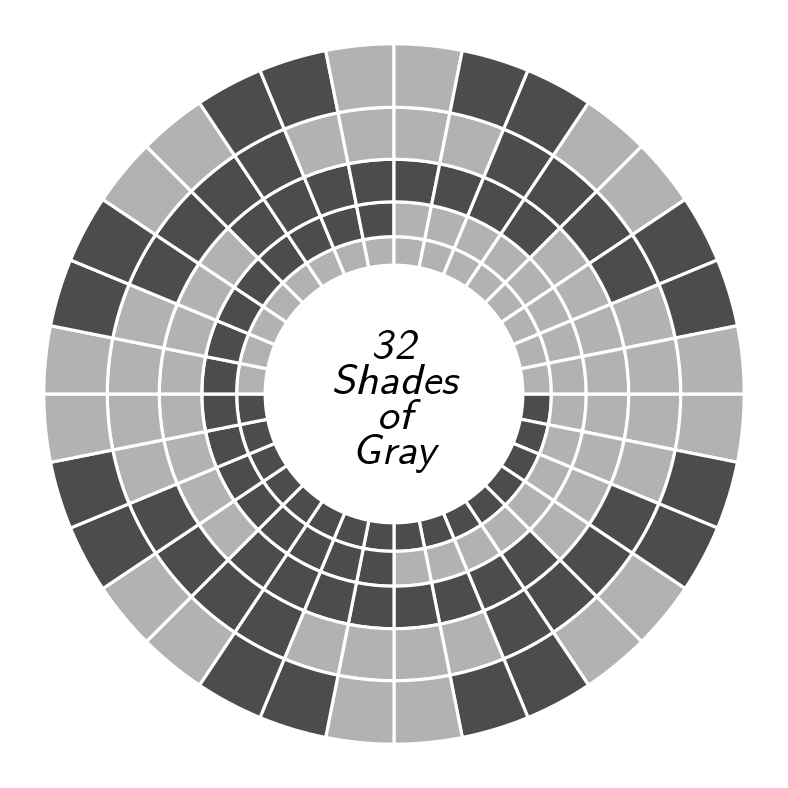# mathr / blog / #Last year I drew some things, and today I recreated one of them using Haskell with the Diagrams library. It features a circular Gray code with five bits, giving 32 possible combinations. A Gray code has the property that neighbouring combinations change only in one position, which is useful for detecting errors in rotary encoders.

Here is the source code:

{-# LANGUAGE FlexibleContexts #-}
{-# LANGUAGE TypeFamilies #-}
import Diagrams.Prelude hiding (size)
import Diagrams.Backend.SVG.CmdLine (B, defaultMain)

main :: IO ()
main = defaultMain diagram

diagram :: Diagram B
diagram
= bg white
. frame 0.125
. centerXY
. mconcat
$[ ring 6 2 1 , ring 5 4 2 , ring 4 8 4 , ring 3 16 8 , ring 2 16 0 , txt ] ring :: Double -> Int -> Int -> Diagram B ring inr count offset = mconcat . zipWith (cell inr) [0..31] . drop offset . cycle$ (  replicate count False
++ replicate count True
)

cell :: Double -> Double -> Bool -> Diagram B
cell inr angle flag
= rotate (angle / 32 @@ turn)
. fc (if flag then dark else light)
. lc white
. translate (r2 (i, 0))
. strokeT
. closeTrail
$mconcat [ p2 (i, 0) ~~ p2 (o, 0) , arc' o h t , rotate t$ p2 (o, 0) ~~ p2 (i, 0)
, arc' i (rotate t h) t'
]
where
t = 1 / 32 @@ turn
t' = -1 / 32 @@ turn
i = size inr
o = size (inr + 1)
h = direction (r2 (1, 0))

light, dark :: Colour Double
light = sRGB 0.7 0.7 0.7
dark  = sRGB 0.3 0.3 0.3

size :: Double -> Double
size r = exp ((r - 7) / 5)

txt :: Diagram B
txt
= scale 0.125
. centerXY
. vcat
$centerXY . atop (translateY 0.4$ strutY 0.8)
. font "LMSans10"
. italic
. fontSizeL 1
. text
<\$> words "32 Shades of Gray"

I had some frustrations until I realized that fontSizeL was what I needed to make the text change size when rendering at different sizes.